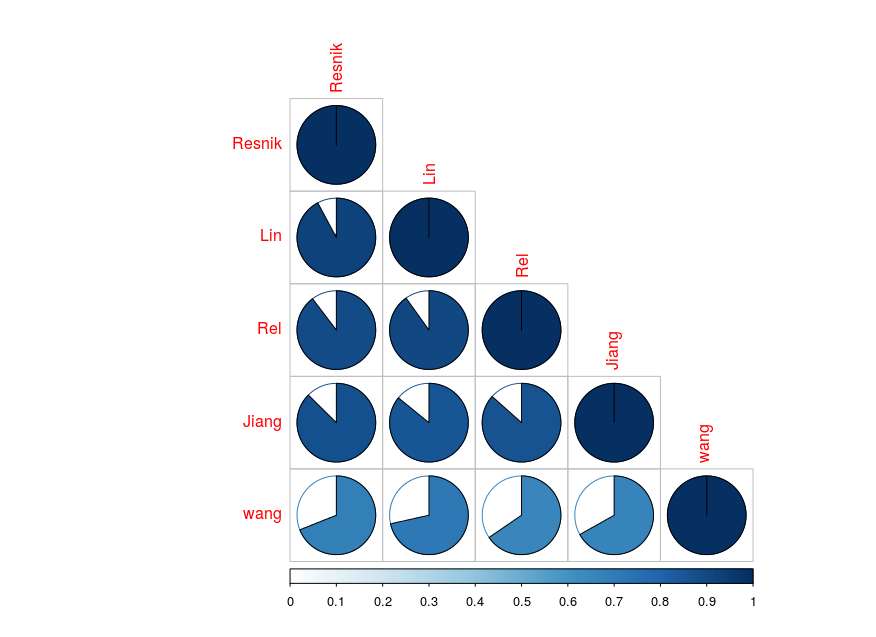# Introduction

In the overview (see`utils::vignette("overview", package ="ViSEAGO")`), we explained how to use ViSEAGO package. In this vignette we explain how to explore the effect of the GO semantic similarity algorithms on the tree structure, and the effect of the trees clustering based on the mouse_bioconductor vignette dataset (see `utils::vignette("2_mouse_bioconductor", package ="ViSEAGO")`).

# Data

Vignette build convenience (for less build time and size) need that data were pre-calculated (provided by the package), and that illustrations were not interactive.

``````# load vignette data
data(
myGOs,
package="ViSEAGO"
)``````

# 1 Clusters-heatmap of GO terms

The GO annotations of genes created and enriched GO terms are combined using `ViSEAGO::build_GO_SS`. The Semantic Similarity (SS) between enriched GO terms are calculated using `ViSEAGO::compute_SS_distances` method. We compute all distances methods with Resnik, Lin, Rel, Jiang, and Wang algorithms implemented in the GOSemSim package . The built object `myGOs` contains all informations of enriched GO terms and the SS distances between them.

Then, a hierarchical clustering method using `ViSEAGO::GOterms_heatmap` is performed based on each SS distance between the enriched GO terms using the `ward.D2` aggregation criteria. Clusters of enriched GO terms are obtained by cutting branches off the dendrogram. Here, we choose a dynamic branch cutting method based on the shape of clusters using dynamicTreeCut [2,3].

``````# compute Semantic Similarity (SS)
myGOs<-ViSEAGO::compute_SS_distances(
myGOs,
distance=c("Resnik","Lin","Rel","Jiang","Wang")
)``````
1. Resnik distance
``````# GO terms heatmap
Resnik_clusters_wardD2<-ViSEAGO::GOterms_heatmap(
myGOs,
showIC=TRUE,
showGOlabels=TRUE,
GO.tree=list(
tree=list(
distance="Resnik",
aggreg.method="ward.D2"
),
cut=list(
dynamic=list(
deepSplit=2,
minClusterSize =2
)
)
),
samples.tree=NULL
)``````
1. Lin distance
``````# GO terms heatmap
Lin_clusters_wardD2<-ViSEAGO::GOterms_heatmap(
myGOs,
showIC=TRUE,
showGOlabels=TRUE,
GO.tree=list(
tree=list(
distance="Lin",
aggreg.method="ward.D2"
),
cut=list(
dynamic=list(
deepSplit=2,
minClusterSize =2
)
)
),
samples.tree=NULL
)``````
1. Rel distance
``````# GO terms heatmap
Rel_clusters_wardD2<-ViSEAGO::GOterms_heatmap(
myGOs,
showIC=TRUE,
showGOlabels=TRUE,
GO.tree=list(
tree=list(
distance="Rel",
aggreg.method="ward.D2"
),
cut=list(
dynamic=list(
deepSplit=2,
minClusterSize =2
)
)
),
samples.tree=NULL
)``````
1. Jiang distance
``````# GO terms heatmap
Jiang_clusters_wardD2<-ViSEAGO::GOterms_heatmap(
myGOs,
showIC=TRUE,
showGOlabels=TRUE,
GO.tree=list(
tree=list(
distance="Jiang",
aggreg.method="ward.D2"
),
cut=list(
dynamic=list(
deepSplit=2,
minClusterSize =2
)
)
),
samples.tree=NULL
)``````
1. Wang distance
``````# GO terms heatmap
Wang_clusters_wardD2<-ViSEAGO::GOterms_heatmap(
myGOs,
showIC=TRUE,
showGOlabels=TRUE,
GO.tree=list(
tree=list(
distance="Wang",
aggreg.method="ward.D2"
),
cut=list(
dynamic=list(
deepSplit=2,
minClusterSize =2
)
)
),
samples.tree=NULL
)``````

# 2 Trees comparison

## 2.1 Global trees comparisons

The dendextend package , offers a set of functions for extending dendrogram objects in R, letting you visualize and compare trees of hierarchical clusterings (see `utils::vignette("introduction", package ="dendextend")`). In this package we use `dendextend::dendlist` and `dendextend::cor.dendlist` functions in order to calculate a correlation matrix between trees, which is based on the Baker Gamma and cophenetic correlation as mentioned in dendextend.

The correlation matrix can be visualized with the nice `corrplot::corrplot` function from corrplot package .

``````# build the list of trees
dend<- dendextend::dendlist(
"Resnik"=slot(Resnik_clusters_wardD2,"dendrograms")\$GO,
"Lin"=slot(Lin_clusters_wardD2,"dendrograms")\$GO,
"Rel"=slot(Rel_clusters_wardD2,"dendrograms")\$GO,
"Jiang"=slot(Jiang_clusters_wardD2,"dendrograms")\$GO,
"Wang"=slot(Wang_clusters_wardD2,"dendrograms")\$GO
)

# build the trees matrix correlation
dend_cor<-dendextend::cor.dendlist(dend)``````
``````# corrplot
corrplot::corrplot(
dend_cor,
"pie",
"lower",
is.corr=FALSEALSE,
cl.lim=c(0,1)
)``````As expected, we can easily tells us that GO semantic similarity algorithms based on the Information Content (IC-based) with Resnik, Lin, Rel, and Jiang methods are more similar than the Wang method which in based on the topology of the GO graph structure (Graph-based).

## 2.2 Paired trees comparison

We can also compare the dendrograms build with, for example, the Resnik and the Wang algorithms using `dendextend::dendlist`, `dendextend::untangle`, and `dendextend::tanglegram` functions. The quality of the alignment of the two trees can be calculated with `dendextend::entanglement` (0: good to 1:bad).

``````# dendrogram list
dl<-dendextend::dendlist(
slot(Resnik_clusters_wardD2,"dendrograms")\$GO,
slot(Wang_clusters_wardD2,"dendrograms")\$GO
)

# untangle the trees (efficient but very highly time consuming)
tangle<-dendextend::untangle(
dl,
"step2side"
)

# display the entanglement
dendextend::entanglement(tangle) # 0.08362968

# display the tanglegram
dendextend::tanglegram(
tangle,
margin_inner=5,
edge.lwd=1,
lwd = 1,
lab.cex=0.8,
columns_width = c(5,2,5),
common_subtrees_color_lines=FALSE
)``````